# 4.2 Units

ArtiSynth is primarily “unitless”, in the sense that it does not define default units for the fundamental physical quantities of time, length, and mass. Although time is generally understood to be in seconds, and often declared as such in method arguments and return values, there is no hard requirement that it be interpreted as seconds. There are no assumptions at all regarding length and mass. Some components may have default parameter values that reflect a particular choice of units, such as MechModel’s default gravity value of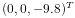, which is associated with the MKS system, but these values can always be overridden by the application.

Nevertheless, it is important, and up to the application developer to ensure, that units be consistent. For example, if one decides to switch length units from meters to centimeters (a common choice), then all units involving length will have to be scaled appropriately. For example, density, whose fundamental units are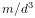, where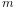is mass and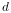is distance, needs to be scaled by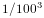, or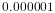, when converting from meters to centimeters.

Table 4.1 lists a number of common physical quantities used in ArtiSynth, along with their associated fundamental units.

unit fundamental units
time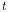distancemassvelocity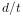acceleration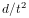force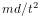work/energy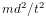torquesame as energy (somewhat counter intuitive)
angular velocity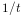angular acceleration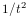rotational inertia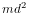pressure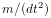Young’s modulusPoisson’s ratio 1 no units; it is a ratio
densitylinear stiffness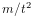linear damping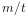rotary stiffnesssame as torque
rotary damping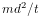mass dampingused in FemModel
stiffness dampingused in FemModel
Table 4.1: Physical quantities and their representation in terms of the fundamental units of mass (), distance (), and time ().

## 4.2.1 Scaling units

For convenience, many ArtiSynth components, including MechModel, implement the interface ScalableUnits, which provides the following methods for scaling mass and distance units:

scaleDistance (s);    // scale distance units by s
scaleMass (s);        // scale mass units by s

A call to one of these methods should cause all physical quantities within the component (and its descendants) to be scaled as required by the fundamental unit relationships as shown in Table 4.1.

Converting a MechModel from meters to centimeters can therefore be easily done by calling

mech.scaleDistance (100);

As an example, adding the following code to the end of the build() method in RigidBodySpring (Section 3.2.2)

System.out.println ("length=" + spring.getLength());
System.out.println ("density=" + box.getDensity());
System.out.println ("gravity=" + mech.getGravity());
mech.scaleDistance (100);
System.out.println ("");
System.out.println ("scaled length=" + spring.getLength());
System.out.println ("scaled density=" + box.getDensity());
System.out.println ("scaled gravity=" + mech.getGravity());

will scale the distance units by 100 and print the values of various quantities before and after scaling. The resulting output is:

length=0.5
density=20.0
gravity=0.0 0.0 -9.8
scaled length=50.0
scaled density=2.0E-5
scaled gravity=0.0 0.0 -980.

It is important not to confuse scaling units with scaling the actual geometry or mass. Scaling units should change all physical quantities so that the simulated behavior of the model remains unchanged. If the distance-scaled version of RigidBodySpring shown above is run, it should behave exactly the same as the non-scaled version.